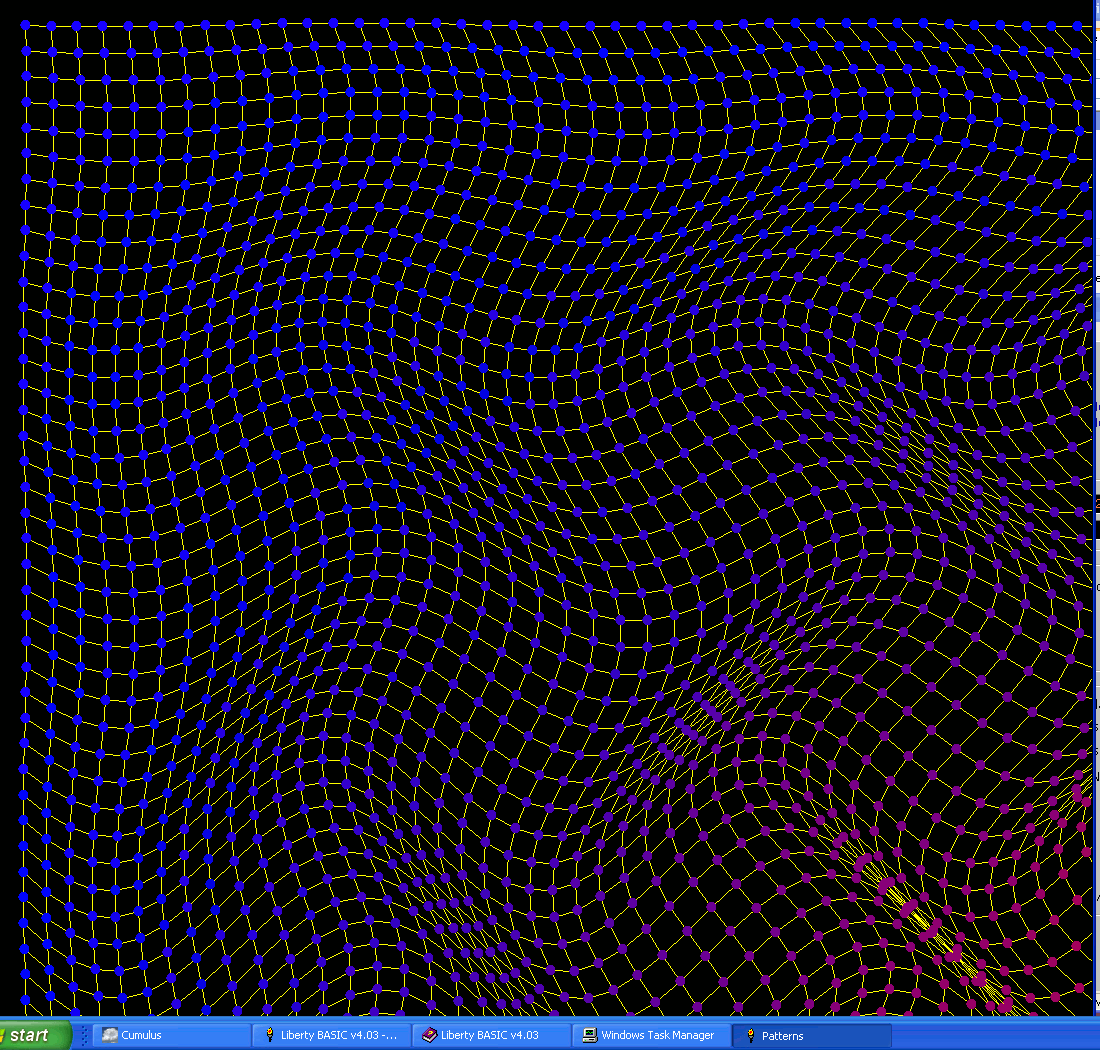# Geometrically distorted gridsThis is just one example of the fun to be had if you distort a rectangular grid systematically. Not dissimilar to some of the Mauritz Escher pictures like 'Night into Day'.

In this example the ( x, y) coordinates of grid points are created by adding sinusoidal terms to the coordinates of the point above or to the left, for the two axes.

You also get great effects by colouring points based on some function of x, y.

```
nomainwin

dim dxy\$( 50, 50)

for x =0 to 50: dxy\$( x, 0) =str\$( x *20); ",0": next x
for y =0 to 50: dxy\$( 0, y) ="0,"; str\$( y *20): next y

for x =1 to 50
for y =1 to 50
dx   =25 +2 *sin( y /3)
dy   =25 +2 *sin( x /3 )
newx =val( word\$( dxy\$( x -1, y), 1, ",")) +dx
newy =val( word\$( dxy\$( x, y -1), 2, ",")) +dy
dxy\$( x, y) =str\$( newx); ","; str\$( newy)
next y
print
next x

WindowWidth  =1100
WindowHeight =1080
UpperLeftX   =  20
UpperLeftY   =  20

open "Patterns" for graphics_nsb_nf as #w

#w "trapclose [quit]"
#w "down ; size 1 ; fill black ; color white"

for x =1 to 49
for y =1 to 49
xs =val( word\$( dxy\$( x, y), 1, ","))
ys =val( word\$( dxy\$( x, y), 2, ","))

#w "size 1 ; color yellow"
#w "place "; xs; " "; ys

xs2 =val( word\$( dxy\$( x+1, y), 1, ","))
ys2 =val( word\$( dxy\$( x+1, y), 2, ","))
#w "goto ";  xs2; " "; ys2

xs3 =val( word\$( dxy\$( x, y+1), 1, ","))
ys3 =val( word\$( dxy\$( x, y+1), 2, ","))
#w "place "; xs;  " "; ys
#w "goto ";  xs3; " "; ys3

#w "size 10 ; color "; x *y /10; " 0 "; 255 -x *y /10
#w "place "; xs; " "; ys
#w "set ";   xs; " "; ys
next y
print
next x

#w "getbmp screen 0 0 1100 1050"
bmpsave "screen", "R:\screen.bmp"

wait

[quit]
close #w
end

```

A second version moves each data point from its rectangular positionm while the first one increments each position sinusoidally from the previous one.```
nomainwin

dim dxy\$( 50, 50)

for x =0 to 50
for y =0 to 50
dx   =40 *cos( y /3)
dy   =10 *sin( x /1.5 )

newx =x *20 +dx
newy =y *20 +dy
dxy\$( x, y) =str\$( newx); ","; str\$( newy)
next y
print
next x

WindowWidth  =1100
WindowHeight =1080
UpperLeftX   =  20
UpperLeftY   =  20

open "Patterns" for graphics_nsb_nf as #w

#w "trapclose [quit]"
#w "down ; size 1 ; fill black ; color white"

for x =1 to 49
for y =1 to 49
xs =val( word\$( dxy\$( x, y), 1, ","))
ys =val( word\$( dxy\$( x, y), 2, ","))

#w "size 1 ; color yellow"
#w "place "; xs; " "; ys

xs2 =val( word\$( dxy\$( x+1, y), 1, ","))
ys2 =val( word\$( dxy\$( x+1, y), 2, ","))
#w "goto ";  xs2; " "; ys2

xs3 =val( word\$( dxy\$( x, y+1), 1, ","))
ys3 =val( word\$( dxy\$( x, y+1), 2, ","))
#w "place "; xs;  " "; ys
#w "goto ";  xs3; " "; ys3

#w "size 12 ; color "; x *y /10; " 0 "; 255 -x *y /10
#w "place "; xs; " "; ys
#w "set ";   xs; " "; ys
next y
print
next x

#w "flush"
#w "getbmp screen 0 0 1100 1050"
filedialog "Save image as", "*.bmp", fn\$
bmpsave "screen", fn\$